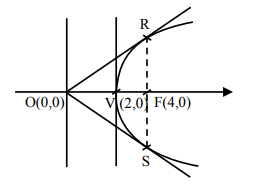# Let a parabola P be such that its vertex`
Question:

Let a parabola P be such that its vertex and focus lie on the positive $x$-axis at a distance 2 and 4 units from the origin, respectively. If tangents are drawn from $\mathrm{O}(0,0)$ to the parabola $\mathrm{P}$ which meet $\mathrm{P}$ at $\mathrm{S}$ and $R$, then the area (in sq. units) of $\Delta S O R$ is equal to :

1. $16 \sqrt{2}$

2. 16

3. 32

4. $8 \sqrt{2}$

Correct Option: , 2

Solution:Clearly RS is latus-rectum

$\because \mathrm{VF}=2=\mathrm{a}$

$\therefore \mathrm{RS}=4 \mathrm{a}=8$

Now $\mathrm{OF}=2 \mathrm{a}=4$

$\Rightarrow$ Area of triangle ORS $=16$